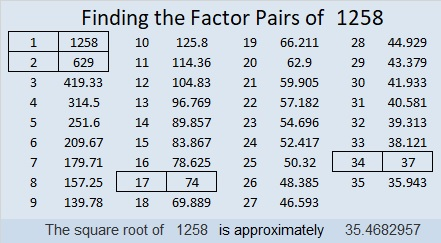# 1258 Mystery Level

This mystery level puzzle might start off easy enough, but before too long it will surely be a mystery what your next step should be. Don’t worry, logic can still lead the way on every step, but finding the logic might be trickier than usual.Print the puzzles or type the solution in this excel file: 12 factors 1251-1258

Here’s some information about the number 1258:

• 1258 is a composite number.
• Prime factorization: 1258 = 2 × 17 × 37
• The exponents in the prime factorization are 1, 1, and 1. Adding one to each and multiplying we get (1 + 1)(1 + 1)(1 + 1) = 2 × 2 × 2 = 8. Therefore 1258 has exactly 8 factors.
• Factors of 1258: 1, 2, 17, 34, 37, 74, 629, 1258
• Factor pairs: 1258 = 1 × 1258, 2 × 629, 17 × 74, or 34 × 37
• 1258 has no square factors that allow its square root to be simplified. √1258 ≈ 35.46831258 is the sum of two squares in two different ways:
27² + 23² = 1258
33² + 13² = 1258

1258 is the hypotenuse of FOUR Pythagorean triples:
200-1242-1258
408-1190-1258
592-1110-1258
858-920-1258